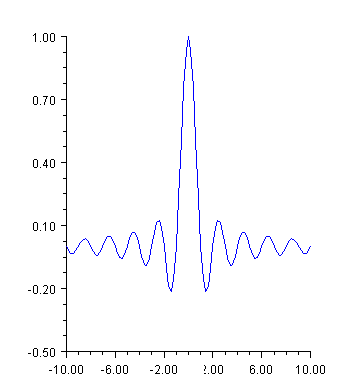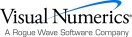## Function Plot

A function plot shows the value of a function f(x) over an interval [a,b]. The function must be defined as an implementation of the ChartFunction interface. A Data node constructor creates a line chart from the function. The look of the function is controlled by the line attributes.

The ChartFunction interface requires that the function name be "F", that the function has a single double argument and that it returns a double.

### Example

This example plots the sinc function on the interval [-10,10]. The sinc function is defined to be sin(πx)/πx. In this example, Sinc is a class that implements  ChartFunction. This is required by the function Data constructor. In the code, the case x = 0 is handled specially to avoid returning NaN.using Imsl.Chart2D;

using System;

using System.Drawing;

public class SampleFunction : FrameChart {

public SampleFunction() {

Chart chart = this.Chart;

AxisXY axis = new AxisXY(chart);

Data data = new Data(axis, new sinc(), -10.0, 10.0);

data.LineColor = Color.Blue;

}

public static void Main(string[] argv) {

System.Windows.Forms.Application.Run(new SampleFunction());

}

}

class sinc : ChartFunction {

public double F(double x) {

if (x == 0.0) return 1.0;

return Math.Sin(Math.PI*x) / (Math.PI*x);

}

}

### Histogram Example

For another example of a ChartFunction, see Histogram.

### Spline Chart

This example shows raw data points, as markers, and their fit to a shape preserving spline. The spline is computed using CsShape found in the IMSL C# Numerical Library Math namespace (which extends Spline). The ChartSpline class wraps the Spline into a ChartFunction. This example also enables the Legend.using Imsl.Chart2D;

using Imsl.Math;

using System.Drawing;

public class SampleSpline : FrameChart {

public SampleSpline() {

Chart chart = this.Chart;

AxisXY axis = new AxisXY(chart);

chart.Legend.IsVisible = true;

double[] x = {0, 1, 2, 3, 4, 5, 8, 9, 10};

double[] y = {1.0, 0.8, 2.4, 3.1, 4.5, 5.8, 6.2, 4.9, 3.7};

Data dataMarker = new Data(axis, x, y);

dataMarker.SetTitle("Data");

dataMarker.DataType = Data.DATA_TYPE_MARKER;

dataMarker.MarkerType = Data.MARKER_TYPE_FILLED_CIRCLE;

CsShape spline = new CsShape(x, y);

Data dataSpline = new Data(axis, new ChartSpline(spline),

0.0, 10.0);

dataSpline.SetTitle("Fit");

dataSpline.LineColor = Color.Blue;

}

public static void Main(string[] argv) {

System.Windows.Forms.Application.Run(new SampleSpline());

}

}Visual Numerics - Developers of IMSL and PV-WAVEhttp://www.vni.com/PHONE: 713.784.3131FAX:713.781.9260